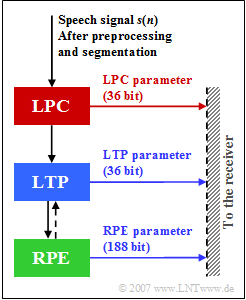# Exercise 3.4Z: GSM Full-Rate Voice CodecLPC, LTP and RPE parameters in the GSM Full-Rate Vocoder

This codec called "GSM Full-Rate Vocoder"  (which was standardized for the GSM system in 1991)  stands for a joint realization of coder and decoder and combines three methods for the compression of speech signals:

• Linear Predictive Coding  $\rm (LPC)$,
• Long Term Prediction  $\rm (LTP)$, and
• Regular Pulse Excitation  $\rm (RPE)$.

The numbers shown in the graphic indicate the number of bits generated by the three units of this Full-Rate speech codec per frame of  $20$  millisecond duration each.

It should be noted that LTP and RPE, unlike LPC, do not work frame by frame, but with sub-blocks of  $5$  milliseconds.  However, this has no influence on solving the task.

The input signal in the above graphic is the digitalized speech signal  $s_{\rm R}(n)$.

This results from the analog speech signal  $s(t)$  by

• a suitable limitation to the bandwidth $B$,
• sampling at the sampling rate $f_{\rm A} = 8 \ \rm kHz$,
• quantization with $13 \ \rm bit$,
• following segmentation into blocks of each $20 \ \rm ms$.

The further tasks of preprocessing will not be discussed in detail here.

Notes:

### Questionnaire

1

To which bandwidth $B$  must the speech signal be limited?

 $B \ = \$ $\ \rm kHz$

2

Of how many samples  $(N_{\rm R})$  is there a speech frame?  How large is the input data rate $R_{\rm In}$?

 $N_{\rm R} \hspace{0.18cm} = \$ $\ \rm samples$ $R_{\rm In} \hspace{0.15cm} = \$ $\ \rm kbit/s$

3

What is the output data rate $R_{\rm Out}$ of the GSM–full rate codec?

 $R_{\rm Out} \ = \$ $\ \rm kbit/s$

4

Which statements apply to the block "LPC"?

 LPC makes a short-term prediction over one millisecond. The  $36$  LPC bits specify coefficients that the receiver uses to undo the LPC filtering. The filter for short-term prediction is recursive. The LPC output signal is identical to the input signal  $s_{\rm R}(t)$.

5

Which statements regarding the block "LTP" are true?

 LTP removes periodic structures of the speech signal. The long-term prediction is performed once per frame. The memory of the LTP predictor is up to  $15 \ \rm ms$.

6

Which statements apply to the block "RPE"?

 RPE delivers fewer bits than LPC and LTP. RPE removes unimportant parts for the subjective impression. RPE subdivides each sub-block into four sub-sequences. RPE selects the sub-sequence with the minimum energy.

### Solution

#### Solution

(1)  To satisfy the sampling theorem, the bandwidth $B$  must not exceed  $f_{\rm A}/2 \hspace{0.15cm}\underline{= 4 \ \ \rm kHz}$.

(2)  The given sampling rate  $f_{\rm A} = 8 \ \rm kHz$  results in a distance between individual samples of  $T_{\rm A} = 0.125 \ \rm ms$.

• Thus a speech frame of  $20 {\rm ms}$  consists of  $N_{\rm R} = 20/0.125 = \underline{160 \ \rm samples}$, each quantized with  $13 \ \rm bit$.
• The data rate is thus
$$R_{\rm In} = \frac{160 \cdot 13}{20 \,{\rm ms}} \hspace{0.15cm} \underline {= 104\,{\rm kbit/s}}\hspace{0.05cm}.$$

(3)  The graph shows that per speech frame  $36 \ {\rm (LPC)} + 36 \ {\rm (LTP)} + 188 \ {\rm (RPE)} = 260 \ \ \rm bit$  are output.

• From this the output data rate is calculated as
$$R_{\rm Out} = \frac{260}{20 \,{\rm ms}} \hspace{0.15cm} \underline {= 13\,{\rm kbit/s}}\hspace{0.05cm}.$$
• The compression factor achieved by the full-rate speech codec is thus  $104/13 = 8$.

(4)  The first two statements are true:

• The 36 LPC bits describe a total of eight filter coefficients of a non-recursive filter, whereby eight  $\rm ACF$ values are determined from the short-term analysis and where these are converted into reflection factors  $r_{k}$  after the so-called "Schur recursion".
• From these the eight LAR coefficients are calculated according to the function  ${\rm ln}\big[(1 - r_{k})/(1 + r_{k})\big]$, quantized with a different number of bits and sent to the receiver.
• The LPC output signal has a significantly lower amplitude than its input  $s_{\rm R}(n)$, and it has a significantly reduced dynamic range and a flatter spectrum.

(5)  Correct are the statements 1 and 3, but not the second:

• The LTP analysis and filtering is done blockwise every  $5 \ \rm ms$  ⇒   $(40$  samples$)$, i.e. four times per speech frame.
• The cross correlation function  $\rm (CCF)$  between the current sub-block and the three previous sub-blocks is formed.
• For each sub-block, an LTP delay and an LTP gain are determined which best match the sub-block.
• A correction signal of the following component "RPE" is also taken into account.
• For the long-term prediction, as with the LPC, the output is reduced in redundancy compared to the input.

(6)  The statements 2 and 3 are correct:

• The fact that statement 1 is wrong can be seen from the graphic on the data page, because  $188$  of the  $260$  output bits come from the RPE.  Voice would be understandable with RPE alone (without LPC and LTP).
• Regarding the last statement:  The RPE is of course looking for the subsequence with the maximum energy.  The RPE pulses are a subsequence  $(13$  of  $40$  samples$)$  of three bits per subframe of  $5 \ \rm ms$  and accordingly  $12$  bits per  $20 \ \rm ms$  frame.
• The "RPE pulse" thus occupies  $13 \cdot 12 = 156$  of the  $260$  output bits.

More details about the RPE block can be found on the page  RPE coding  of the book  "Examples of Communication Systems".